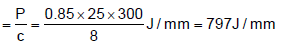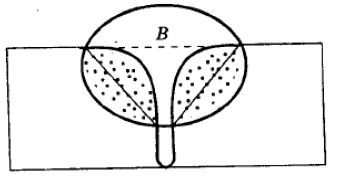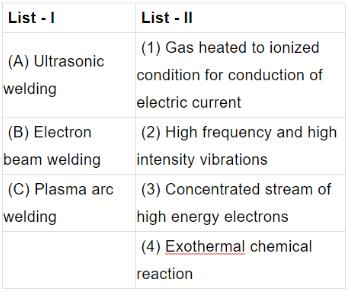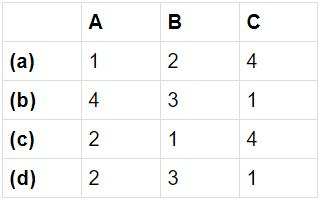Courses

# Test: Fabrication Processes (Welding) - 2

## 30 Questions MCQ Test RRB JE for Mechanical Engineering | Test: Fabrication Processes (Welding) - 2

Description
This mock test of Test: Fabrication Processes (Welding) - 2 for Mechanical Engineering helps you for every Mechanical Engineering entrance exam. This contains 30 Multiple Choice Questions for Mechanical Engineering Test: Fabrication Processes (Welding) - 2 (mcq) to study with solutions a complete question bank. The solved questions answers in this Test: Fabrication Processes (Welding) - 2 quiz give you a good mix of easy questions and tough questions. Mechanical Engineering students definitely take this Test: Fabrication Processes (Welding) - 2 exercise for a better result in the exam. You can find other Test: Fabrication Processes (Welding) - 2 extra questions, long questions & short questions for Mechanical Engineering on EduRev as well by searching above.
QUESTION: 1

Solution:
QUESTION: 2

Solution:
QUESTION: 3

### By which one of the following methods gray iron is usually welded?

Solution:
QUESTION: 4

Preheating before welding is done to

Solution:
QUESTION: 5

In a gas welding of mild steel using an oxy-acetylene flame, the total amount of acetylene consumed is 10 litre. The oxygen consumption from the cylinder is

Solution:
QUESTION: 6

In arc welding, d.c. reverse polarity is used to hear greater advantage in

Solution:
QUESTION: 7

In an arc welding process, the voltage and current are 25 V and 300 A respectively. The arc heat transfer efficiency is 0.85 and welding speed is 8 mm/see. The net heat input (in J/mm) is

Solution:

Effective heat transfer per second (P) = η VI =0.85x25x300 J/s

Speed (c) = 8 mm/s

Net heat inputQUESTION: 8

Arc blow is more common in

Solution:
QUESTION: 9

An arc welded joint is shown in the above figure. The part labelled 'B' in the figure is known asSolution:
QUESTION: 10

In which of the following welding processes, flux is used in the form of granules?

Solution:
QUESTION: 11

In resistance welding, heat is generated due to the resistance between

Solution:
QUESTION: 12

Assertion (A): Spot welding is adopted to weld two overlapped metal pieces between two electrode points. Reason (R): In this process when current is switched on, the lapped pieces of metal are heated in a restricted area.

Solution:
QUESTION: 13

Two 1 mm thick steel sheets are to be spot welded at a current of 5000 A. Assuming effective resistance to be 200 micro-ohms and current flow time of 0.2 second, heat generated during the process will be

Solution:

Q = I2Rt = 50002 × (200 ×10−6 ) × 0.2J = 1000J

QUESTION: 14

Assertion (A): In electron beam welding process, vacuum is an essential process parameter

Reason (R): Vacuum provides a highly efficient shield on weld zone

Solution:

Explanation : EBW is carried out in vacuum to avoid dispersion or deviation from the focus.

QUESTION: 15

Match List I (Welding processes) with List II (Features) and select the correct answer using the codes given below the Lists:Codes:Solution:
QUESTION: 16

Which one of the following is a solid state joining process?

Solution:
QUESTION: 17

The strength of a brazed joint

Solution:
QUESTION: 18

Consider the following statements: The magnitude of residual stresses in welding depends upon

1. design of weldment

2. support and clamping of components

3. welding process used

4. amount of metal melted / deposited

Which of the statements given above are correct?

Solution:

Explanation : Residual stress depends on welding parameters such as current, voltage, speed, current.

QUESTION: 19

The ratio of acetylene to oxygen is approximately………. for a neutral flames used in gas welding.

Solution:
QUESTION: 20

In Oxyacetylene gas welding, temperature at the inner cone of the flame is around

Solution:
QUESTION: 21

In oxy-acetylene gas welding, for complete combustion, the volume of oxygen required per unit of acetylene is

Solution:
QUESTION: 22

Oxyacetylene reducing flame is used while carrying out the welding on

Solution:
QUESTION: 23

Assertion (A): The electrodes of ac arc welding are coated with sodium silicate, whereas electrodes used for dc arc welding are coated with potassium silicate binders.

Reason (R): Potassium has a lower ionization potential than sodium.

Solution:
QUESTION: 24

In d.c. welding, the straight polarity (electrode negative) results in

Solution:
*Multiple options can be correct
QUESTION: 25

Which one of the following statements is correct?

Solution:

Explanation : Although the classic flux for steel was borax, and the filler rod is dipped in a can of borax, while the rod is still very hot. The shielding gas is supplied by the torch itself as the work is done inside the area covered by the flame.

So the flux took care of minor oxide contamination, and the torch flame kept the weld puddle from seeing atmospheric oxygen.

Generally DCSP is used in GTAW with the exception of AC being used in aluminium and Magnesium.

QUESTION: 26

In MIG welding, the metal is transferred into the form of which one of the following?

Solution:
QUESTION: 27

For butt -welding 40 mm thick steel plates, when the expected quantity of such jobs is 5000 per month over a period of 10 year, choose the best suitable welding process out of the following available alternatives.

Solution:

We need maximum metal deposition rate so choice SAW.
Note: You know maximum metal deposition rate of all welding is SAW and it may joint 40 mm thick plates easily.

QUESTION: 28

Resistance spot welding is performed on two plates of 1.5 mm thickness with 6 mm diameter electrode, using 15000 A current for a time duration of 0.25 seconds. Assuming the interface resistance to be 0.0001 Ω , the heat generated to form the weld is

Solution:

H = I2Rt = 150002 × 0.0001× 0.25J = 5625 W − s

QUESTION: 29

Which one of the following is not an electric resistance method of welding?

Solution:
QUESTION: 30

In which one of the following welding techniques is vacuum environment required?

Solution: